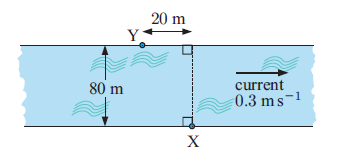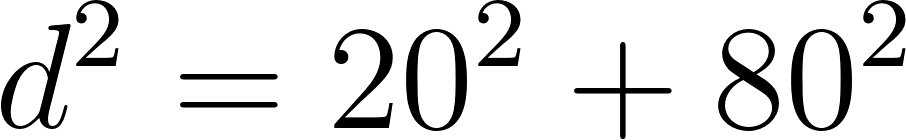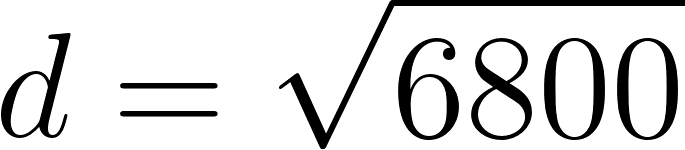×
Get Full Access to Mathematics For The International Student: Mathematics Sl - 3 Edition - Chapter 13 - Problem 4
Get Full Access to Mathematics For The International Student: Mathematics Sl - 3 Edition - Chapter 13 - Problem 4

×

# As part of an endurance race, Stephanie needs to swim from X to Y across a wide riverISBN: 9781921972089 446

## Solution for problem 4 Chapter 13

Mathematics for the International Student: Mathematics SL | 3rd Edition

• Textbook Solutions
• 2901 Step-by-step solutions solved by professors and subject experts
• Get 24/7 help from StudySoup virtual teaching assistantsMathematics for the International Student: Mathematics SL | 3rd Edition

4 5 1 414 Reviews
12
4
Problem 4

As part of an endurance race, Stephanie needs to swim from X to Y across a wide river. Stephanie swims at 1:8 m s1 in still water. The river flows with a consistent current of 0:3 m s1 as shown. a Find the distance from X to Y. b In which direction should Stephanie aim so that the current will push her onto a path directly towards Y? c Find the time Stephanie will take to cross the river

Step-by-Step Solution:

Problem 4

As part of an endurance race, Stephanie needs to swim from X to Y across a wide river. Stephanie swims at 1:8 m s1 in still water. The river flows with a consistent current of 0:3 m s1 as shown.

a Find the distance from X to Y. ?

b In which direction should Stephanie aim so that the current will push her onto a path directly towards Y?

c Find the time Stephanie will take to cross the river?Step-by-solution

Step 1of  4

a) Consider the figure,The distance from X to Y is d

By pythagoras theorem ,That isSo the distance from X to Y is 82.5 meters.

Step 2 of 4

Step 3 of 4

##### ISBN: 9781921972089

The full step-by-step solution to problem: 4 from chapter: 13 was answered by , our top Math solution expert on 03/15/18, 06:04PM. Mathematics for the International Student: Mathematics SL was written by and is associated to the ISBN: 9781921972089. The answer to “As part of an endurance race, Stephanie needs to swim from X to Y across a wide river. Stephanie swims at 1:8 m s1 in still water. The river flows with a consistent current of 0:3 m s1 as shown. a Find the distance from X to Y. b In which direction should Stephanie aim so that the current will push her onto a path directly towards Y? c Find the time Stephanie will take to cross the river” is broken down into a number of easy to follow steps, and 79 words. This full solution covers the following key subjects: . This expansive textbook survival guide covers 25 chapters, and 453 solutions. This textbook survival guide was created for the textbook: Mathematics for the International Student: Mathematics SL, edition: 3. Since the solution to 4 from 13 chapter was answered, more than 2380 students have viewed the full step-by-step answer.

Unlock Textbook Solution